In :
:ext QuasiQuotes
import qualified H.Prelude as H
H.initialize H.defaultConfig
[r| require("ggplot2")
require("pracma")|]

Loading required package: ggplot2
0x00007f19a800ab30

## Small Dataset for Ringo¶

### Haskell / R Logistics and Functions¶

In :
[r|
ggplotRegression <<- function(the.title, dat, xvar, yvar){

require(ggplot2)

fit <- lm(yvar~xvar, dat)

ggplot(fit$model, aes_string(x = names(fit$model), y = names(fit$model))) + geom_point(size=2, shape=21) + ggtitle(the.title) + theme(plot.title = element_text(hjust = 0.5)) + theme(plot.caption = element_text(hjust = 0.5)) + theme(text=element_text(family="Ariel", size=12)) + stat_smooth(method = "lm", col = "red", se=FALSE) + labs( caption = paste("RSq = ",signif(summary(fit)$r.squared, 5),
"Intercept =",signif(fit$coef[],5 ), " Slope =",signif(fit$coef[], 5),
" P =",signif(summary(fit)$coef[2,4], 5))) } |]  0x00007f19aa787218 ### Ringo Data Exploration¶ #### Let's read in the dataset ..¶ In : [r| dset_small <<- read.table ("TM30-CES99.csv", header=TRUE, sep=","); |]  0x00007f379c186550 #### Now Perform PCA to Yield a 15x15 Translation Matrix¶ In : [r| dset_pca <<-prcomp (dset_small[c(1:15)], center=TRUE, scale=TRUE) |]  0x00007f374dc5ed08 #### Inspect the results¶ In : [rprint| summary(dset_pca) |]  Importance of components: PC1 PC2 PC3 PC4 PC5 PC6 PC7 Standard deviation 3.4971 1.5175 0.67338 0.10657 0.04741 0.01825 0.009159 Proportion of Variance 0.8153 0.1535 0.03023 0.00076 0.00015 0.00002 0.000010 Cumulative Proportion 0.8153 0.9688 0.99906 0.99982 0.99997 0.99999 1.000000 PC8 PC9 PC10 PC11 PC12 PC13 Standard deviation 0.004877 0.003562 0.001035 0.0006333 6.778e-05 3.408e-05 Proportion of Variance 0.000000 0.000000 0.000000 0.0000000 0.000e+00 0.000e+00 Cumulative Proportion 1.000000 1.000000 1.000000 1.0000000 1.000e+00 1.000e+00 PC14 PC15 Standard deviation 3.087e-05 8.541e-06 Proportion of Variance 0.000e+00 0.000e+00 Cumulative Proportion 1.000e+00 1.000e+00 #### PC1 and PC2 have about 97% of the Variance¶ #### Now Export the PCA Rotation Data for HPEC and PSO ("$rotation")¶

In :
[r| x=dset_pca$x[,1] #PC1 y=dset_pca$x[,2] #PC2
z=dset_pca$x[,3] #PC3 dset_small$XScaled = scale(dset_small$X) actX=dset_small$XScaled
exportFrame.x <<- data.frame(x,y,z,actX)
write.csv(exportFrame.x, "frameX.txt", row.names=FALSE) |]

0x00007f3794011760
In :
[r| PC1=dset_pca$x[,1] #PC1 PC2=dset_pca$x[,2] #PC2
z=dset_pca$x[,3] #PC3 actY = scale(dset_small$Y)
exportFrame.y <<- data.frame(PC1,PC2,actY)
write.csv(exportFrame.y, "frameY.txt", row.names=FALSE) |]

0x00007f3794011760
In :
[r| PC1=dset_pca$x[,1] #PC1 PC2=dset_pca$x[,2] #PC2
z=dset_pca$x[,3] #PC3 actZ = scale(dset_small$Z)
exportFrame.z <<- data.frame(PC1,PC2,actZ)
write.csv(exportFrame.z, "frameZ.txt", row.names=FALSE) |]

0x00007f3794011760

#### HP Evolutionary Stack Computer (HPEC) Results for X¶

For now it's a bit kludgy. A 'csv' file is created by a Haskell program from a copy/paste from the Scheme HPEC program and read in here. V1 is the predicted value, V2 is the actual value. The plot below is just the first sanity check for the process.

In :
[rprint| predictedX <<- read.csv(file = 'paste01.csv', header=FALSE) |]

             V1          V2
1   1.967583586  1.91722730
2   0.184061967  0.23260496
3  -1.349531851 -1.20956200
4   0.460303492  0.53274115
5  -0.470423178 -0.40758297
6  -0.692009319 -0.57130696
7  -0.807577964 -0.75788250
8  -0.885254003 -0.81346742
9  -1.448396390 -1.30273622
10  1.361707391  1.18267670
11 -0.096817288 -0.07728859
12  0.415490229  0.34516248
13 -0.903047587 -0.85893738
14  0.660921239  0.61212317
15  0.615241395  0.50023070
16 -0.746647860 -0.70874320
17 -0.731379402 -0.65534474
18 -0.399388294 -0.40413144
19  0.657257843  0.54011049
20  0.561231068  0.32381517
21  2.225716834  1.88229399
22  1.440051321  1.15469987
23  2.523094183  2.23224846
24  2.554034201  2.15196176
25  0.640818223  0.47358627
26  2.542991374  2.05724150
27  0.595546582  0.45258449
28 -1.104526341 -1.02683786
29  2.092765299  1.60232781
30 -0.021590592 -0.12022656
31  2.283934775  1.74259233
32  0.824825397  0.55661999
33  2.325155318  1.88001417
34 -0.179547049 -0.28404900
35 -1.109725727 -1.02787118
36  1.182469887  1.04004366
37 -0.938209793 -0.87482595
38  1.836054631  1.47247315
39 -1.308537831 -1.19643531
40 -0.800019080 -0.80077264
41  1.973246788  1.72808122
42  0.107452546 -0.13086817
43  0.405689935  0.06406313
44 -1.464484749 -1.31376423
45 -0.532422790 -0.62398883
46 -0.053554138 -0.17644076
47  0.219156777  0.10008642
48  0.664082288  0.43206477
49 -1.037602319 -1.01469619
50 -0.712153400 -0.72789940
51 -0.504071509 -0.54482462
52 -1.200569966 -1.15913581
53 -1.008452381 -1.02767149
54  1.587848040  1.46723734
55  1.215979153  1.09944236
56 -0.013662831 -0.09071530
57 -0.751200547 -0.76091699
58 -0.918461151 -0.87769558
59  1.601094685  1.47179650
60  1.899364949  1.78493910
61  0.656793073  0.61452745
62 -0.006625292  0.03428158
63 -1.339702517 -1.19978514
64 -0.728358344 -0.64043005
65 -1.280783956 -1.12700664
66 -1.200964373 -1.06134530
67 -1.114886439 -0.96790961
68 -0.315532445 -0.22108964
69 -0.733133262 -0.64061953
70  0.158190505  0.32340881
71 -1.396853882 -1.20680616
72  0.706697832  0.72843177
73 -0.765244451 -0.49441142
74 -1.480529333 -1.31845621
75 -1.437290200 -1.22807777
76 -1.080956530 -0.76002903
77 -1.138330594 -0.90389231
78 -1.207075638 -0.87423986
79  0.420000695  0.60499951
80 -0.110415963  0.14338849
81 -1.106109245 -0.79917737
82  1.814162854  1.82554571
83  0.422552457  0.60882165
84 -1.461506230 -1.30760515
85 -1.150748700 -0.97554135
86 -0.002716820  0.23851140
87 -1.158696259 -0.93473319
88 -0.112596628  0.10196405
89 -1.199920093 -0.97673257
90 -0.978641214 -0.76231674
91  1.185101379  1.13173222
92 -0.431611441 -0.31199658
93  0.199598821  0.29017108
94 -1.101196660 -0.91644545
95  0.512469004  0.56565983
96  1.191682738  1.21983827
97 -0.601630534 -0.41681415
98  0.294530015  0.40035822
99 -0.355628398 -0.27264993
In :
[rgraph|
ggplot(predictedX, aes(x=predictedX[,2], y=predictedX[,1])) +
ggtitle("Actual X vs Predicted X") +
xlab("Actual") + ylab("Predicted") +
geom_point(size=2, shape=21) +
theme(plot.title = element_text(hjust = 0.5)) +
theme(text=element_text(family="Ariel", size=12)) |]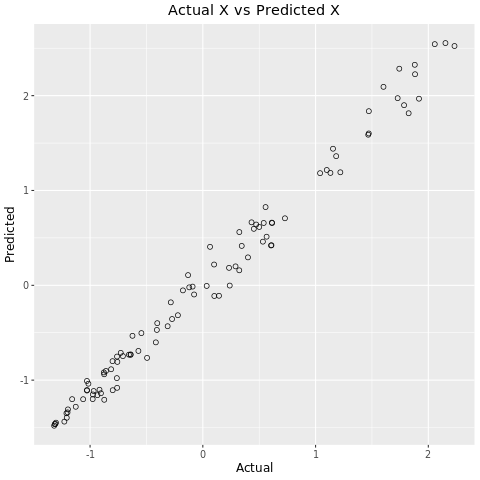#### With This Formuls (Raw)¶

To interpret this, it helps to know that,

• push (0) - means put constant 1
• push (1) - means put constant 5
• push (2) - means put constant PC1
• push (3) - means put constant PC2

I'll have to check this to be absolutely sure ..

• Didn't use PC3
• It trained on all the data, no hidden data
• Need to get the 'round trip', i.e.generate model and test on new data
• Try detrending?
• Is it accurate enough .. No way to tell until the data is remapped to actual X from PCA space.
• Need to try Y, and Z

#### Another Formula from HP Stack Machine¶

Another run of the HP stack computer using PC1, PC2, and PC3 was conducted yielding the following formula,

$Prediction = 0.25 \cdot (P2 - P1 - P3)$

This seems a bit better,

$R^2 = 0.987$

In :
[rgraph|
pc1 = exportFrame.x$x pc2 = exportFrame.x$y
pc3 = exportFrame.x$z actualB <<- exportFrame.x$actX
predictionB <<- 0.26 * (pc2 - pc1 - pc3)
newFrame = data.frame (actualB, predictionB)

ggplot(newFrame, aes(x=newFrame[,1], y=newFrame[,2])) +
ggtitle("Actual X vs Predicted X") +
xlab("Actual") + ylab("Predicted") +
geom_smooth(method='lm',se=FALSE) +
geom_point(size=2, shape=21) +
theme(plot.title = element_text(hjust = 0.5)) +
theme(text=element_text(family="Ariel", size=12)) |]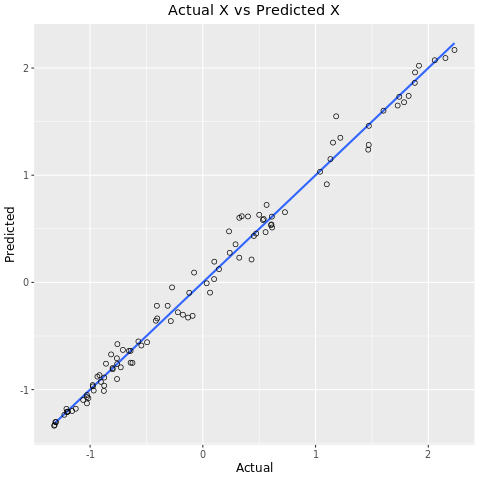In :
[rprint| res = lm(predictionB ~ actualB)
cor(predictionB, actualB)^2 |]

 0.9876915

Note only a small improvement in R squared with the addition of PC3.

#### Predict X Values Using PSO¶

As the formulas seem quite simple, a PSO template was created to predict X values as follows for a prediction $P$ using only PC1 and PC2.

$P = k_1 + k_2 ((k_3\cdot pc_2) - (k_4\cdot pc_1))$

Where $k_1$ through $k_4$ are found using PSO, specifically,

$k_1 = 0.056$, $\ k_2 = 0.27$, $\ k_3 = 0.91$, $\ k_4 = 0.96$

The graphical results are shown below.

In :
[rgraph|
pc1 = exportFrame.x$x pc2 = exportFrame.x$y
actualA = exportFrame.x$actX predictionA = 0.056 + 0.27 * ((0.91*pc2) - (0.96*pc1)) newFrame = data.frame (actualA, predictionA) ggplotRegression("Actual vs Predicted for X", newFrame, actualA, predictionA) |]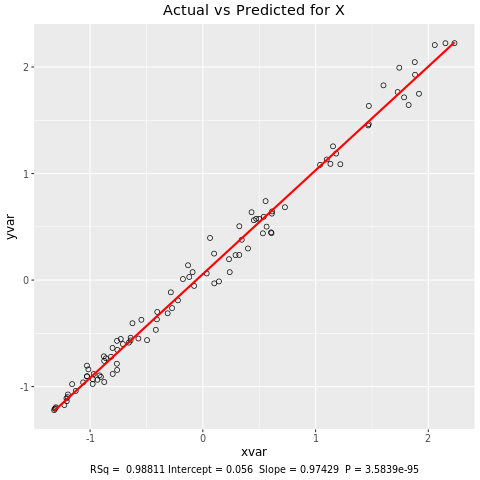#### Predicting Y Values¶ Unfortunately I didn't take good notes but I believe the prediction formula for Y came from HPEC.$P = 5 + -0.26*pc_1 + 0.16*pc_2 + 0.01 *pc_1*pc_2$Graphical results are shown below. In : [rgraph| pc1 = exportFrame.y$PC1
pc2 = exportFrame.y$PC2 actualA <<- exportFrame.y$actY
predictionA <<- -0.26*pc1 + 0.16*pc2 + 0.01 *pc1*pc2 + 5
newFrame <<- data.frame (actualA, predictionA)
ggplotRegression("Actual vs Predicted for Y", newFrame, actualA, predictionA) |]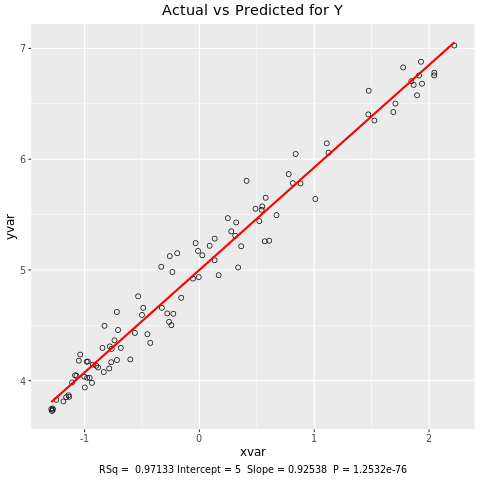#### Predicting Z using PSO¶

Several templates were tried.

A template which allowed non-integer powers of PC1 and PC2 was tried which produced good results in training but horrible results in testing, implying overfitting.

Eventually the same template that was used for X is applied here. Specifically,

$P = k_1 + k_2 ((k_3\cdot pc_2) - (k_4\cdot pc_1))$

Where $k_1$ through $k_4$ are,

$k_1 = -0.17$, $\ k_2 = 0.27$, $\ k_3 = 0.95$, $\ k_4 = 0.75$

Graphically shown below.

In :
[rgraph|
pc1 = exportFrame.z$PC1 pc2 = exportFrame.z$PC2
actualA <<- exportFrame.z$actZ predictionA <<- -0.17 - 0.18 * ((1.43*pc2) - (-1.13*pc1)) predictionA <<- -0.17 + 1.15 * ((-0.24*(pc2**0.1859)) - (1.23*(pc1**0.4397))) predictionA <<- -0.17 + 0.27 * ((-0.95*pc2) - (0.75*pc1)) newFrame <<- data.frame (actualA, predictionA) ggplotRegression("Actual vs Predicted for Z", newFrame, actualA, predictionA) |]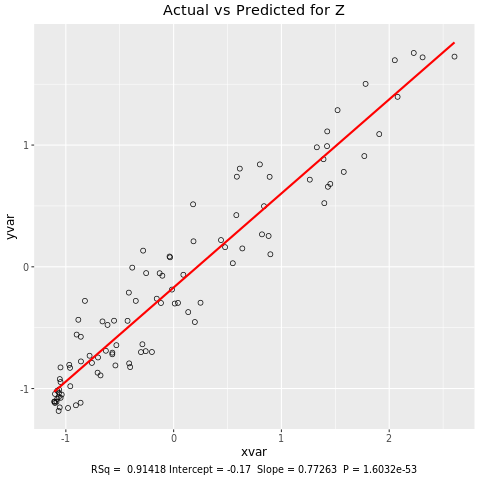#### Poor Z Performance¶ Z's relatively poor performace is of interest. If it is noise a Histogram plot of the$R^2$residuals might give a clue to it's source. This is done below. In : [rgraph| fit <- lm(predictionA ~ actualA, newFrame) g <- fit$residuals
h <- hist(g,main="Histogram of Z Residuals", breaks=11,family="ariel",col="lightgray")
xfit <- seq(min(g), max(g), length = 40)
yfit <- dnorm(xfit, mean = mean(g), sd = sd(g))
yfit <- yfit * diff(h$mids[1:2]) * length(g) lines(xfit, yfit, col = "blue", lwd = 1) |]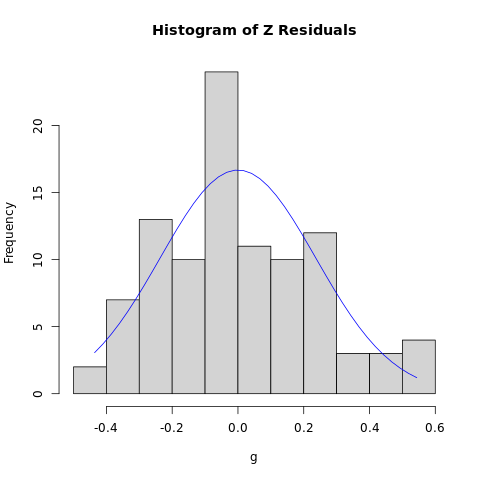The distrubution appears to be somewhat Gaussian. So, what are sources of Gaussian noise (as opposed to Uniform noise)? Johnson–Nyquist noise (or thermal noise) is Gaussian. The implication might be that the signal to noise ratio is worse for the Z values. #### Summary¶ This analysis was intended to be a first cut to determine the difficulty of the prediction problem. For HPEC all the data was used for training and predicting. For PSO half the data was used to train and the full dataset was used for predicting. So, to summarize, Using the small (~100 data samples) dataset reasonable$R^2\$ values were obtained to predict X, Y, and Z values.

Value Predicted R-Squared
X 0.988
Y 0.971
Z 0.914